# NetworkX

One of the powerful library used for graph building activities is NetworkX. It is widely used in solving graph problems and network related queries. Lets have a look into NetworkX now.

In order to use it with python import it,

`import networkx as nx`

The following basic graph types are provided as Python classes:
Graph
This class implements an undirected graph. It ignores multiple edges between two nodes. It does allow
self-loop edges between a node and itself.
DiGraph
Directed graphs, that is, graphs with directed edges. Provides operations common to directed graphs, (a
subclass of Graph).
MultiGraph
A flexible graph class that allows multiple undirected edges between pairs of nodes. The additional
MultiDiGraph
A directed version of a MultiGraph

```g=nx.Graph()
nx.draw(g)```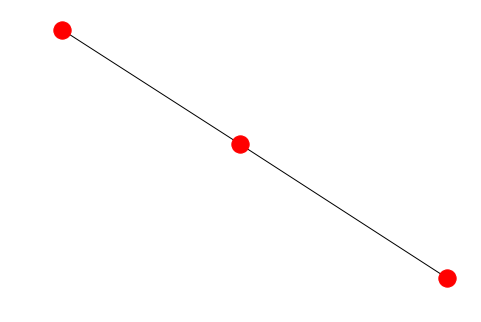we can even make edges or not as per requirement, like the code below:

```import matplotlib.pyplot as plt
g2=nx.Graph()
#nx.draw(g2)

pos = nx.spring_layout(g2)  # compute graph layout
nx.draw(g2, pos, node_size=700)  # draw nodes and edges
nx.draw_networkx_labels(g2, pos)

labels = nx.get_edge_attributes(g2, 'weight')
nx.draw_networkx_edge_labels(g2, pos, edge_labels=labels)

plt.show(g2)```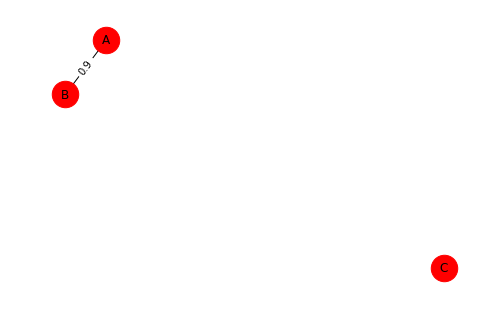Now, if we go for a shortest path algorithm, networkx really rocks, lets take an example;

```G=nx.Graph()
e = [('a', 'b', 0.3), ('b', 'c', 0.9), ('a', 'c', 0.5), ('c', 'd', 1.2)]

pos = nx.spring_layout(G)
nx.draw(G,pos,node_size=700)
nx.draw_networkx_labels(G, pos)

labels = nx.get_edge_attributes(G, 'weight')
nx.draw_networkx_edge_labels(G, pos, edge_labels=labels)

plt.show(G)```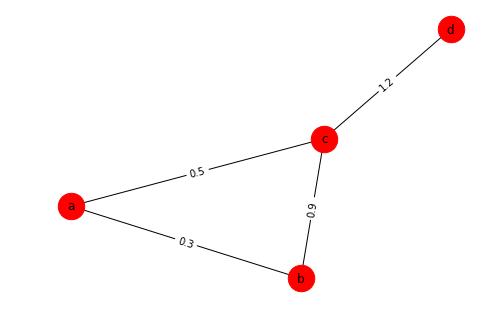`print(nx.dijkstra_path(G, 'a', 'd'))`
`['a', 'c', 'd']`

We can even write few modular code to check the adjacency matrix and number of nodes relationship,

```#Asignment 2 Comparision
for i in range(3):
GraphA=lstA[i]
GraphB=lstB[i]

count=i
count=count+1
print('-------------****************-------------')
print('Number of nodes: ',count*100)
for k in range(2,4):
print('An^k','n',count*100,'k',k)
#print(B_nk)
plt.plot(A_nk)
plt.show()
print('Bn^k','n',count*100,'k',k)
#print(B_nk)
plt.plot(B_nk)
plt.show()
print('------************------')```
```-------------****************-------------
Number of nodes:  100
An^k n 100 k 2```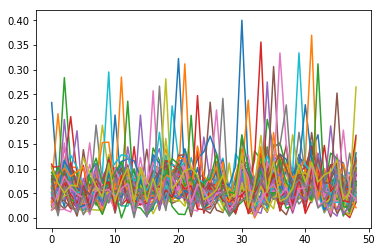`Bn^k n 100 k 2`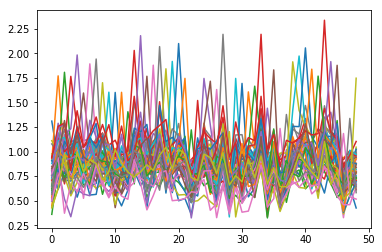•cocomelody

Having read this I thought it was extremely informative.
I appreciate you finding the time and energy to put this
information together. I once again find myself personally spending a
lot of time both reading and commenting. But so what, it
was still worthwhile!

•oprolevorter

Somebody necessarily assist to make critically articles I would state. This is the very first time I frequented your website page and up to now? I amazed with the research you made to make this actual post incredible. Magnificent activity!

•coca

Its like you read my mind! You appear to know